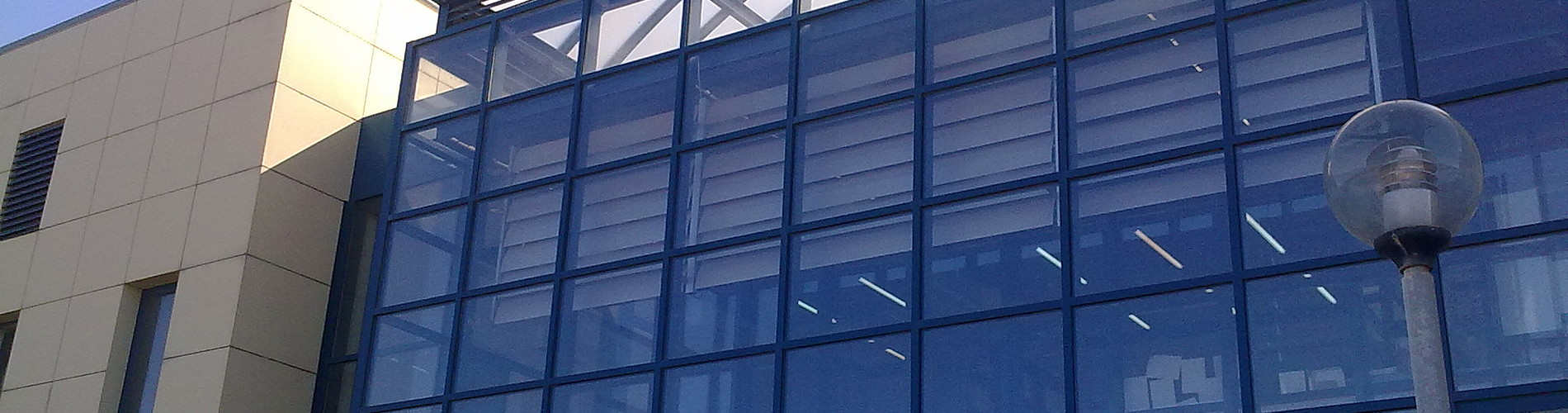School of Chemical and Environmental Engineering

Now offering two distinct diplomas: Chemical Engineering and Environmental Engineering

# Ordinary differential equations

1. COURSE INFORMATION:

 School Chemical and Environmental Engineering Course Level Undergraduate Direction - Course ID MATH 203 Semester 4th Course Category Required Course Modules Instruction Hours per Week ECTS Lectures Tutorials and Lab exercises 5 Th=3, E=1, L=1 4 Course Type Scientific area Prerequisites Instruction/Exam Language Greek The course is offered to Erasmus students No Course URL https//www.eclass.tuc.gr/courses/MHPER310/   (in Greek)

2. LEARNING OUTCOMES

 Learning Outcomes In many problems of the everyday life we deal with the change of a quantity, of our interest, in relation to time (rate of change). Differential equations are the proper tool used for (mathematical) modeling and solving these problems. The aim of the course is to introduce (i) basic concepts of differential equations and (ii) techniques for solving o.d.e.’s (iii) some of their applications in basic problems of Engineering, electromagnetism, the Environment, etc.Upon successful completion of the course, the students will be able to:Recognizes the different types of differential equationsSolve differential equations.Interprets the solutions of differential equations.Uses differential equations to model natural phenomena. General Competencies/Skills Work autonomouslywork in teams Retrieve, analyze and synthesize data and information, with the use of proper technologies.advance free, creative and causative thinking.

3. COURSE SYLLABUS

 Introduction to Ordinary Differential Equations (definition of an O.D.E, order, degree, solution of an O.D.E. e.t.c.)Ordinary Differential Equations of 1st order: separation of variables, homogenous.Ordinary Differential Equations of 1st order: Bernoulli, Riccati, Euler, exact o.d.e’s.Method of integrating factor. 1st order linear differential equations.Applications of 1st order o.d.e.’s to problems in Chemical and Environmental Engineering.Linear dependence/ independence condition, Wroskian, the y = gY transformation.Linear differential equations of higher order with constant coefficients: homogenous.Linear differential equations of higher order with constant coefficients: non homogenous.Linear differential equations of higher order with non constant coefficients.Laplace’ s transformation method.Applications of higher order O.D.E in Engineering and electricity.Power series method.Systems of differential equations with constant coefficients.

4. INSTRUCTION and LEARNING METHODS - ASSESSMENT

 Lecture Method Direct (face to face) Use of Information and Communication Technology Specialized software, Power point presentations, E-class support Instruction Organisation Activity Workload per Semester (hours) - Lectures 39 - Tutorials 13 - Software applications in statistics 13 - Autonomous study 35 Course Total 100 Assessment Method Ι. Written final examination (100%). Theoretical problems to be resolved. II. Problems/Exercises during the semester (Bonus 10%). ORI. Midterm exams (100%=50%+50%)II. Problems/Exercises during the semester (Bonus 10%).